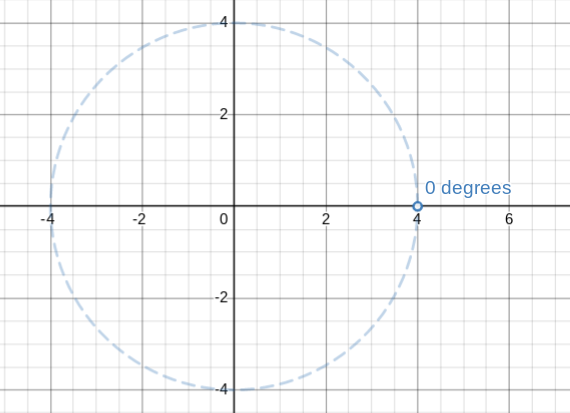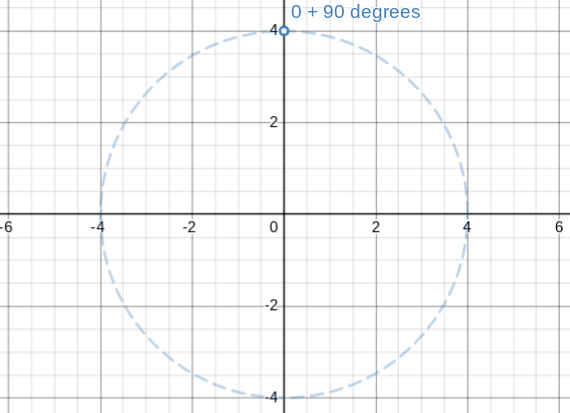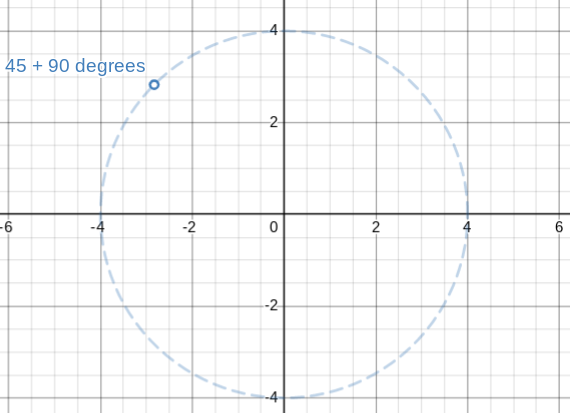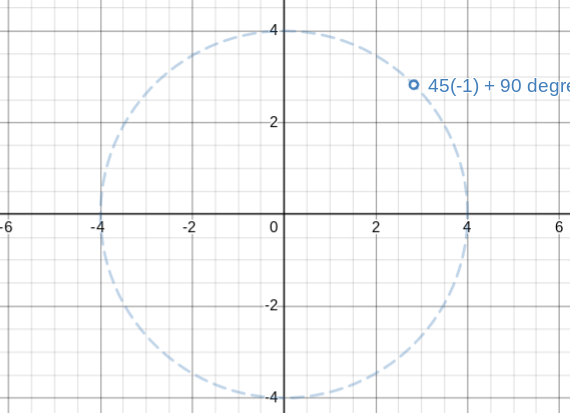# Why is angles going counter clockwise

``````local RADIUS = 0.5
local ANGLE_OFFSET = 90

-- createprompt

for i = 1, TotalButtons do
local Angle = (360 / TotalButtons) * (i - 1)

print(i, Angle)
self:CreatePrompt(PromptData, i, Angle)
end
``````

the print prints correctly
1 0
2 90
3 180
4 270
Yet they displaying opposite direction. 2 and 4 should swap positions1 Like

This math is working exactly as it should. Your positioning UI to points on a circle because your using math.cos and math.sin. And I can see that because there is 4 prompts, it’s positioning it on vertexes on a square, because a square has 4 sides.

Usually, 0 degrees starts at the right side of the circle:But because of the angle offset you added, the start is here:As you can see, adding more degrees caused the point to rotate around the circle counterclockwise. This is normal. If you want counterclockwise, just multiply the degrees (but not with the offset) by -1.

With and without negative degrees:So to fix your problem: Multiply angle by -1.

The UI looks good by the way!

2 Likes

This topic was automatically closed 14 days after the last reply. New replies are no longer allowed.